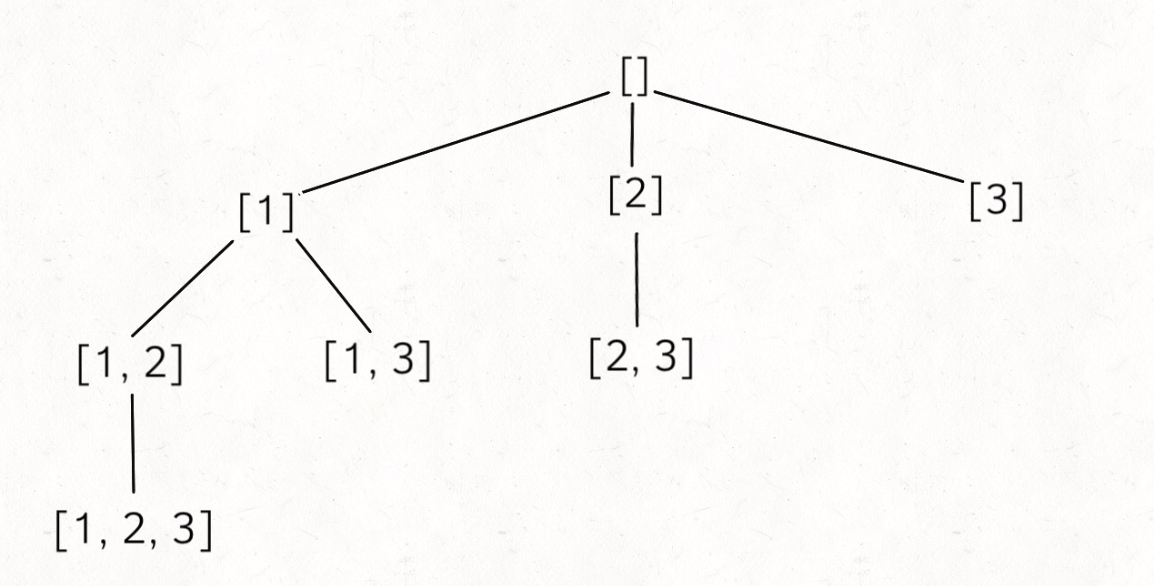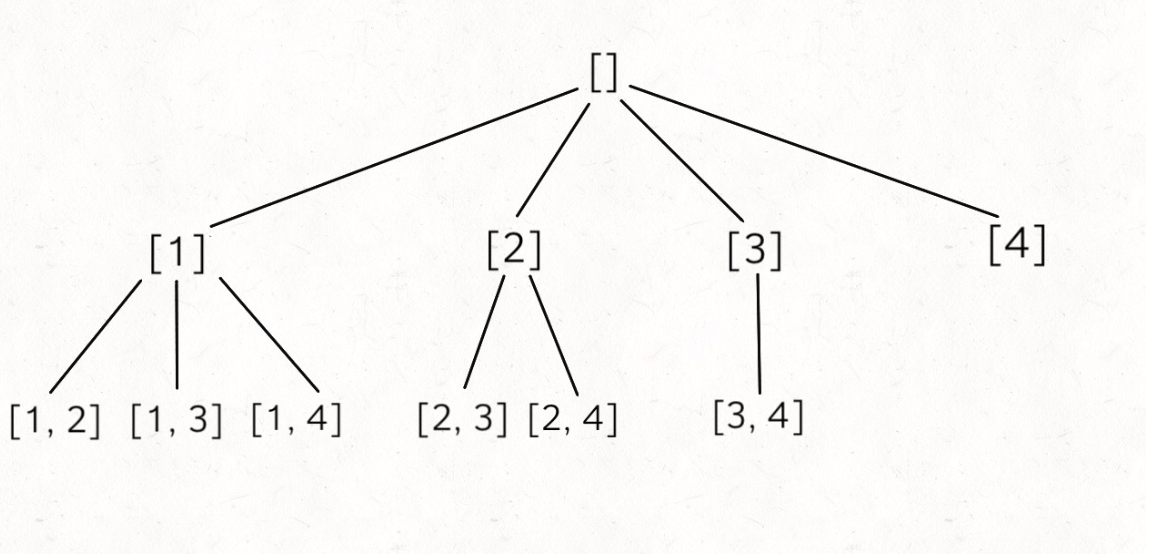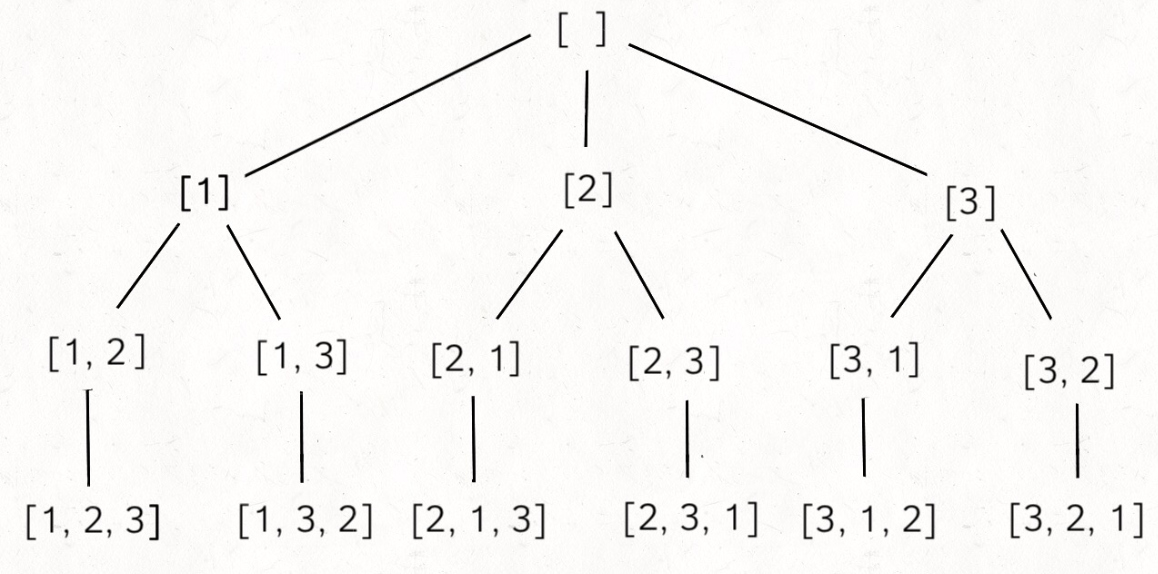• 累计撰写 270 篇文章
• 累计创建 6 个标签
• 累计收到 1 条评论

### 目 录CONTENT# 回溯算法团灭子集、排列、组合问题.md2021-08-22 / 0 评论 / 0 点赞 / 12 阅读 / 5,829 字 / 正在检测是否收录...

## 回溯算法团灭子集、排列、组合问题

输入: nums = [1,2,3]

[
,
,
,
[1,2,3],
[1,3],
[2,3],
[1,2],
[]
]


[ [],,,[1,2] ]


subset([1,2,3]) - subset([1,2])
= ,[1,3],[2,3],[1,2,3]


subset([1,2,3])
= A + [A[i].add(3) for i = 1..len(A)]


vector<vector<int>> subsets(vector<int>& nums) {
// base case，返回一个空集
if (nums.empty())
return {{}};

// 把最后一个元素拿出来
int n = nums.back();
nums.pop_back();

// 先递归算出前面元素的所有子集
vector<vector<int>> res = subsets(nums);

int size = res.size();
for (int i = 0; i < size; i++)
{
// 然后在之前的结果之上追加
res.push_back(res[i]);

//在每个结果后面push一个前面保存的元素n
res.back().push_back(n);
//eg:{1,2} : {},{1},{2},{1,2}
//n = 2  res = subsets()
//     n = 1    res = sunsets()
//              res = { {} }
//	   i = 0; i < 1;i ++
//     res = { {}, {} }
//	   res = { {},{1} }
//i = 0;i < 2;i ++
//res = { {},{1},{} }
//res = { {},{1},{2} }
//res = { {},{1},{2},{1} }
//res = { {},{1},{2},{1,2} }
}

return res;
}


for (int i = 0; i < size; i++) {
res.push_back(res[i]); // O(N)
res.back().push_back(n); // O(1)
}


result = []
def backtrack(路径, 选择列表):
if 满足结束条件:
return
for 选择 in 选择列表:
做选择
backtrack(路径, 选择列表)
撤销选择


vector<vector<int>> res;

vector<vector<int>> subsets(vector<int>& nums) {
// 记录走过的路径
vector<int> track;
backtrack(nums, 0, track);
return res;
}

void backtrack(vector<int>& nums, int start, vector<int>& track) {
res.push_back(track);
for (int i = start; i < nums.size(); i++) {
// 做选择
track.push_back(nums[i]);
// 回溯
backtrack(nums, i + 1, track);
// 撤销选择
track.pop_back();
}
}

//eg : {1,2}
//backtrack(nums,0,track);
//res : { {} } i = 0;i < 2;i++
//track : {1};
//		backtrack(i + 1)
//      res = { {},{1}},i = 1;i < 2;i++
//		track : { {1,2} };
//			backtrack(nums,i + 1,track)
//			res : {{},{1},{1,2}};i = 2;i < 2;i++ return;
//		track : {1} return;
//track : { {} }
//track : { {2} };
//		backtrack(i + 1);
//		res : {{},{1},{1,2},{2}};i = 2;i < 2;i++;return
//track : { {} };
//return输入: n = 4, k = 2

[
[2,4],
[3,4],
[2,3],
[1,2],
[1,3],
[1,4],
]vector<vector<int>>res;

vector<vector<int>> combine(int n, int k) {
if (k <= 0 || n <= 0)
return res;

vector<int> track;
backtrack(n, k, 1, track);
return res;
}

void backtrack(int n, int k, int start, vector<int>& track) {
// 到达树的底部
if (k == track.size())
{
res.push_back(track);
return;
}

// 注意 i 从 start 开始递增
for (int i = start; i <= n; i++) {
// 做选择
track.push_back(i);
backtrack(n, k, i + 1, track);
// 撤销选择
track.pop_back();
}
}


backtrack 函数和计算子集的差不多，区别在于，更新 res 的时机是树到达底端时

输入: [1,2,3]

[
[1,2,3],
[1,3,2],
[2,1,3],
[2,3,1],
[3,1,2],
[3,2,1]
]java代码：

List<List<Integer>> res = new LinkedList<>();

/* 主函数，输入一组不重复的数字，返回它们的全排列 */
List<List<Integer>> permute(int[] nums) {
// 记录「路径」
backtrack(nums, track);
return res;
}

void backtrack(int[] nums, LinkedList<Integer> track) {
// 触发结束条件
if (track.size() == nums.length) {
return;
}

for (int i = 0; i < nums.length; i++) {
// 排除不合法的选择
if (track.contains(nums[i]))
continue;
// 做选择
// 进入下一层决策树
backtrack(nums, track);
// 取消选择
track.removeLast();
}
}


C++代码：

class Solution {
public:
vector<vector<int>> ret;//保存最后的结果
vector<vector<int>> permute(vector<int>& nums) {
if(nums.empty())
{
return ret;
}

vector<int> track;//保存单次结果
backtrack(nums,track);//递归
return ret;
}

void backtrack(vector<int>& nums,vector<int>& track)
{
if(track.size() == nums.size())//当track中的元素和nums中的元素个数相等
//时，代表是一种全排列，可以放到ret的结果中
{
ret.push_back(track);
return;
}

//循环回朔
for(int i = 0;i < nums.size();i++)
{
//如果nums[i]这个元素已经存在track中，代表上次的选择错误，
//continue回去，判断下一个元素
//反之，就把nums这个元素直接放到track中，继续回朔判断
if(IsExist(track,nums[i]))
{
track.push_back(nums[i]);
}
else
{
continue;
}

backtrack(nums,track);

//撤销选择
track.pop_back();
}
}

//在track中判断一个元素是否已经存在
bool IsExist(vector<int>& track,int val)
{
for(auto e : track)
{
if(e == val)
{
return false;
}
}
return true;
}
};


C++代码：

class Solution {
public:
vector<string>ret;
//判断结果是否已经在ret当中
bool IsEist(vector<string>& a,string& val)
{
for(auto e : a)
{
if(e == val)
{
return false;
}
}
return true;
}

void backtrack(string& str,int start)
{
//如果当start走到最后一个字符的位置
if(start == str.size() - 1)
{
//如果这次结果已经在ret当中就不添加进去
if(IsEist(ret,str))
ret.push_back(str);
}
else
{
for(int i = start;i < str.size();i++)
{
//做选择
swap(str[i],str[start]);
backtrack(str,start + 1);
//撤回
swap(str[i],str[start]);
}
}
}

vector<string> Permutation(string str) {
if(str.size()< 1)
{
return ret;
}
//str是字符串，0代表从哪里开始
backtrack(str,0);
//按字典排序
sort(ret.begin(),ret.end());
return ret;
}
};


==模版模版模版！！！==

0